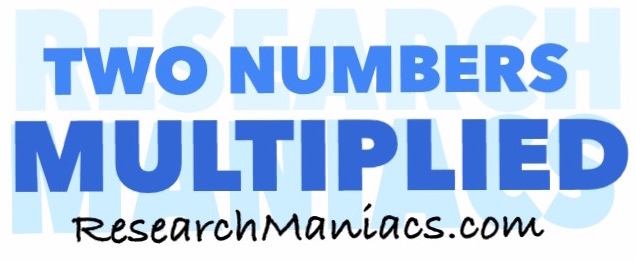What two numbers multiply to 4000?What 2 numbers do you multiply to get 4000? In other words, what number can you multiply with another number to get 4000?

The formula to solve this would be:

X x Y = 4000

There are at least two combinations of two numbers that you can multiply together to get 4000.

For your convenience, we have made a list of all the combinations of two numbers multiplied by each other that will make 4000:

4000 = 1 x 4000
4000 = 2 x 2000
4000 = 4 x 1000
4000 = 5 x 800
4000 = 8 x 500
4000 = 10 x 400
4000 = 16 x 250
4000 = 20 x 200
4000 = 25 x 160
4000 = 32 x 125
4000 = 40 x 100
4000 = 50 x 80
4000 = 80 x 50
4000 = 100 x 40
4000 = 125 x 32
4000 = 160 x 25
4000 = 200 x 20
4000 = 250 x 16
4000 = 400 x 10
4000 = 500 x 8
4000 = 800 x 5
4000 = 1000 x 4
4000 = 2000 x 2
4000 = 4000 x 1

Two Numbers Multiplied
Enter another number below to see which combinations of two numbers multiplied will equal that number.

What two numbers multiply to 4001
Go here for the next number on our list.